The ratio of the magnetic field at the centre of a current carrying circular wire and the magnetic field at the centre of a square coil made from the same length of wire will be

(a) $\frac{{\mathrm{\pi }}^{2}}{4\sqrt{2}}$                             (b) $\frac{{\mathrm{\pi }}^{2}}{8\sqrt{2}}$

(c) $\frac{\mathrm{\pi }}{2\sqrt{2}}$                             (d) $\frac{\mathrm{\pi }}{4\sqrt{2}}$

Concept Questions :-

Magnetic field due to various cases
High Yielding Test Series + Question Bank - NEET 2020

Difficulty Level:

A particle of charge +q and mass m moving under the influence of a uniform electric field $\mathrm{E}\stackrel{^}{\mathrm{i}}$ and a uniform magnetic field $\mathrm{B}\stackrel{^}{\mathrm{k}}$ follows trajectory from P to Q as shown in figure. The velocities at P and Q are $\mathrm{v}\stackrel{^}{\mathrm{i}}$ and $-2\mathrm{v}\stackrel{^}{\mathrm{j}}$ respectively. Which of the following statement(s) is/are correct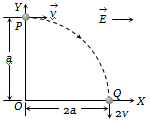(a) $\mathrm{E}=\frac{3}{4}\frac{{\mathrm{mv}}^{2}}{\mathrm{qa}}$

(b) Rate of work done by electric field at P is $\frac{3}{4}\frac{{\mathrm{mv}}^{3}}{\mathrm{a}}$

(c)  Rate of  work done by both fields at Q is zero

(d) All of the above

Concept Questions :-

Lorentz force
High Yielding Test Series + Question Bank - NEET 2020

Difficulty Level:

Figure shows a square loop ABCD with edge length a. The resistance of the wire ABC is r and that of ADC is 2r. The value of magnetic field at the centre of the loop assuming uniform wire is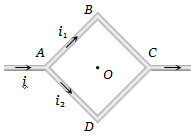(a) $\frac{\sqrt{2}{\mu }_{0}i}{3\mathrm{\pi a}}\odot$                                  (b) $\frac{\sqrt{2}{\mu }_{0}i}{3\mathrm{\pi a}}\otimes$

(c) $\frac{\sqrt{2}{\mu }_{0}i}{\mathrm{\pi a}}\odot$                                   (d) $\frac{\sqrt{2}{\mu }_{0}i}{\mathrm{\pi a}}\otimes$

Concept Questions :-

Magnetic field due to various cases
High Yielding Test Series + Question Bank - NEET 2020

Difficulty Level:

For a positively charged particle moving in a  x- plane initially along the x-axis, there is a sudden change in its path due to the presence of electric and/or magnetic fields beyond P. The curved path is shown in the x-y plane and is found to be non-circular. Which one of the following combinations is possible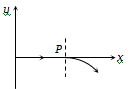(a)                   (b)

(c) $\stackrel{\to }{\mathrm{E}}=0;\stackrel{\to }{\mathrm{B}}=\mathrm{c}\stackrel{^}{\mathrm{j}}+\mathrm{b}\stackrel{^}{\mathrm{k}}$                    (d)  $\stackrel{\to }{\mathrm{E}}=\mathrm{ai};\stackrel{\to }{\mathrm{B}}=\mathrm{c}\stackrel{^}{\mathrm{k}}+\mathrm{b}\stackrel{^}{\mathrm{j}}$

Concept Questions :-

Lorentz force
High Yielding Test Series + Question Bank - NEET 2020

Difficulty Level:

Figure shows the cross-sectional view of the partially hollow cylindrical conductor with inner radius 'R' and outer radius '2R' carrying uniformly distributed current along it's axis. The magnetic induction at point 'P' at a distance $\frac{3R}{2}$ from the axis of the cylinder will be: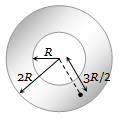1. Zero

2. $\frac{5{\mu }_{0}i}{72\mathrm{\pi R}}$

3. $\frac{7{\mu }_{0}i}{18\mathrm{\pi R}}$

4.  $\frac{5{\mu }_{o}i}{36\mathrm{\pi R}}$

Concept Questions :-

Magnetic field due to various cases
High Yielding Test Series + Question Bank - NEET 2020

Difficulty Level:

A long wire AB is placed on a table. Another wire PQ of mass 1.0 g and length 50 cm is set to slide on two rails PS and QR. A current of 50A is passed through the wires. At what distance above AB, will the wire PQ be in equilibrium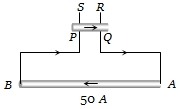(a) 25 mm                           (b) 50 mm

(c) 70 mm                           (d) 100 mm

Concept Questions :-

Lorentz force
High Yielding Test Series + Question Bank - NEET 2020

Difficulty Level:

A particle with charge q, moving with a momentum p, enters a uniform magnetic field normally. The magnetic field has magnitude B and is confined to a region of width d, where $d<\frac{p}{Bq}$. The particle is deflected by an angle $\theta$ in crossing the field, then :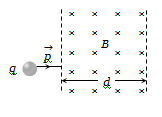(a) $\mathrm{sin}\theta =\frac{Bqd}{p}$                              (b) $\mathrm{sin}\theta =\frac{p}{Bqd}$

(c) $\mathrm{sin}\theta =\frac{Bp}{qd}$                                (d) $\mathrm{sin}\theta =\frac{pd}{Bq}$

Concept Questions :-

Lorentz force
High Yielding Test Series + Question Bank - NEET 2020

Difficulty Level:

The same current i = 2A is flowing in a wireframe as shown in the figure. The frame is a combination of two equilateral triangles ACD and CDE of side 1m. It is placed in uniform magnetic field B = 4T acting perpendicular to the plane of the frame. The magnitude of the magnetic force acting on the frame is: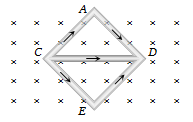1. 24 N                                         2. Zero

3. 16 N                                         4. 8 N

Concept Questions :-

Lorentz force
High Yielding Test Series + Question Bank - NEET 2020

Difficulty Level:

In the given figure net magnetic field at O will be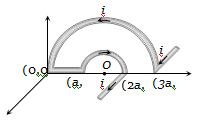(a) $\frac{2{\mu }_{0}i}{3\mathrm{\pi a}}\sqrt{4-{x}^{2}}$                                 (b) $\frac{{\mu }_{0}i}{3\mathrm{\pi a}}\sqrt{4+{x}^{2}}$

(c) $\frac{2{\mu }_{0}i}{3\mathrm{\pi a}}\sqrt{4+{x}^{2}}$                                  (d) $\frac{2{\mu }_{0}i}{3\mathrm{\pi a}}\sqrt{\left(4-{\mathrm{\pi }}^{2}\right)}$

Concept Questions :-

Magnetic field due to various cases
High Yielding Test Series + Question Bank - NEET 2020

Difficulty Level:

In the following figure a wire bent in the form of a regular polygon of n sides is inscribed in a circle of radius a. Net magnetic field at centre will be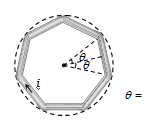(a) $\frac{{\mu }_{o}i}{2\mathrm{\pi a}}\mathrm{tan}\frac{\mathrm{\pi }}{n}$                                                (b) $\frac{{\mu }_{0}ni}{2\mathrm{\pi a}}\mathrm{tan}\frac{\mathrm{\pi }}{n}$

(c)$\frac{2}{\mathrm{\pi }}\frac{ni}{a}{\mu }_{0}\mathrm{tan}\frac{\mathrm{\pi }}{n}$                                             (d) $\frac{ni}{2a}{\mu }_{0}\mathrm{tan}\frac{\mathrm{\pi }}{n}$

Concept Questions :-

Magnetic field due to various cases